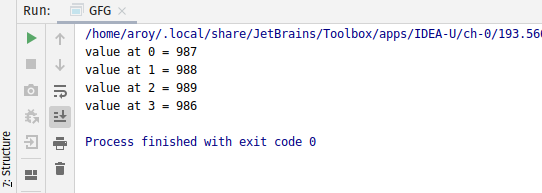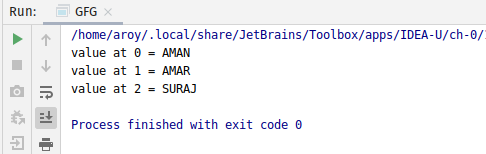Skip to content
Related Articles
AtomicReferenceArray getOpaque() method in Java with Examples
• Last Updated : 02 Jun, 2020

The getOpaque() method of a AtomicReferenceArray class is used to return the value of the element at index i for this AtomicReferenceArray object with memory effects as specified by VarHandle.getOpaque(java.lang.Object…). This VarHandle.getOpaque(java.lang.Object…) method handles operation with no assurance of memory ordering effects with respect to other threads.

Syntax:

```public final E getOpaque(int i)
```

Parameters: This method accepts the index i to get the value.

Return value: This method returns the current value at index i.

Below programs illustrate the getOpaque() method:
Program 1:

 `// Java program to demonstrate``// AtomicReferenceArray.getOpaque() method`` ` `import` `java.util.concurrent.atomic.*;`` ` `public` `class` `GFG {``    ``public` `static` `void` `main(String[] args)``    ``{`` ` `        ``// create an atomic reference array``        ``// object which stores Integer.``        ``AtomicReferenceArray array``            ``= ``new` `AtomicReferenceArray(``5``);`` ` `        ``// set some value in array``        ``array.set(``0``, ``987``);``        ``array.set(``1``, ``988``);``        ``array.set(``2``, ``989``);``        ``array.set(``3``, ``986``);`` ` `        ``// get and print the value``        ``// using getOpaque method``        ``for` `(``int` `i = ``0``; i < ``4``; i++) {`` ` `            ``int` `value = array.getOpaque(i);``            ``System.out.println(``"value at "``                               ``+ i + ``" = "``                               ``+ value);``        ``}``    ``}``}`
Output:Program 2:

 `// Java program to demonstrate``// AtomicReferenceArray.getOpaque() method`` ` `import` `java.util.concurrent.atomic.*;`` ` `public` `class` `GFG {``    ``public` `static` `void` `main(String[] args)``    ``{`` ` `        ``// create a array of Strings``        ``String[] names``            ``= { ``"AMAN"``, ``"AMAR"``, ``"SURAJ"` `};`` ` `        ``// create an atomic reference object.``        ``AtomicReferenceArray array``            ``= ``new` `AtomicReferenceArray(names);`` ` `        ``// get and print the value``        ``// using getOpaque method``        ``for` `(``int` `i = ``0``; i < array.length(); i++) {`` ` `            ``String value = array.getOpaque(i);``            ``System.out.println(``"value at "``                               ``+ i + ``" = "``                               ``+ value);``        ``}``    ``}``}`
Output:Attention reader! Don’t stop learning now. Get hold of all the important Java Foundation and Collections concepts with the Fundamentals of Java and Java Collections Course at a student-friendly price and become industry ready. To complete your preparation from learning a language to DS Algo and many more,  please refer Complete Interview Preparation Course.

My Personal Notes arrow_drop_up